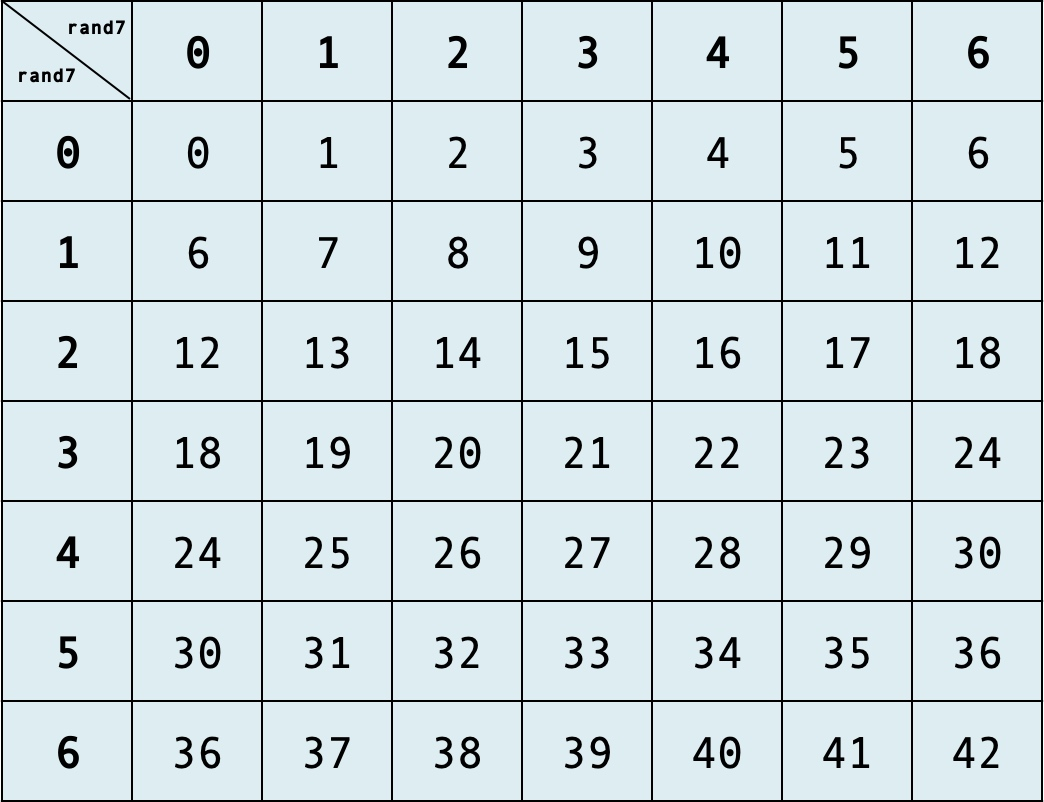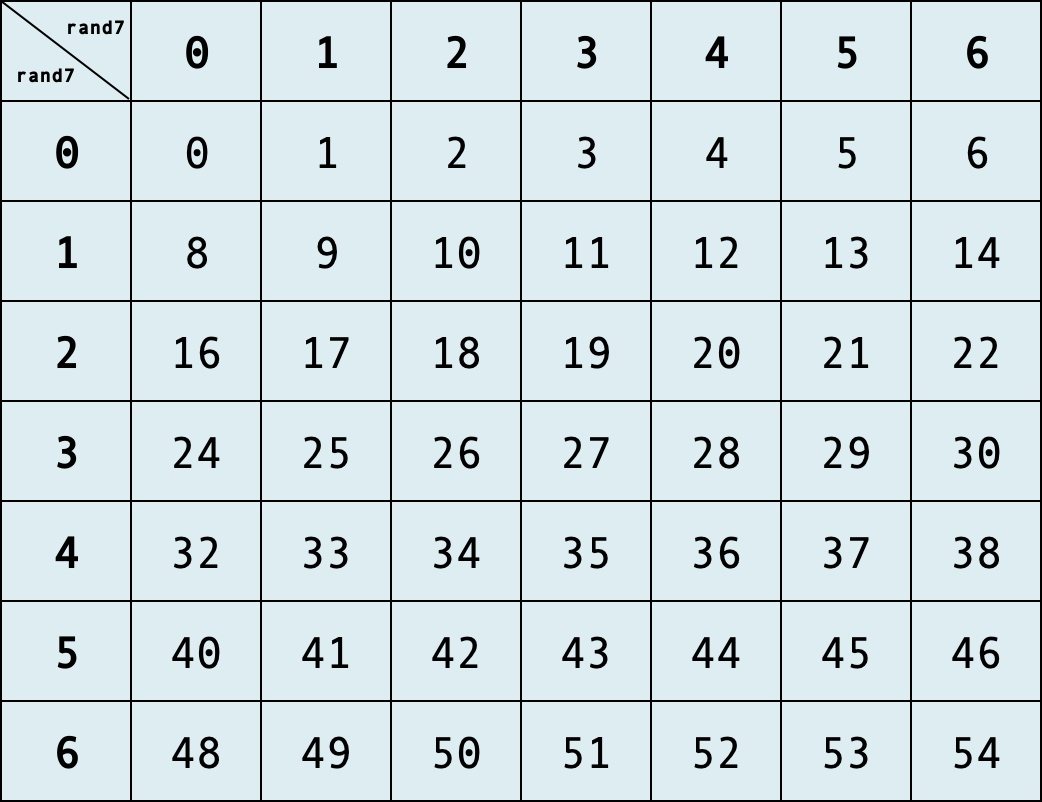## 引言

1. 有一枚不均匀的硬币，要求产生均匀的概率分布
2. 有一枚均匀的硬币，要求产生不均匀的概率分布，如 0.25 和 0.75
3. 利用 Rand7() 实现 Rand10()

## 不均匀硬币，产生等概率

// 不均匀硬币，返回 0、1 的概率分别为 0.6、0.4
int coin() {
return (rand() % 10) > 5;
}


0 0.6*0.6=0.36 0.6*0.4=0.24
1 0.4*0.6=0.24 0.4*0.4=0.16

int coin_new() {
while (true) {
int a = coin();
if (coin() != a) return a;
}
}


#include <iostream>
#include <map>
using namespace std;

// 不均匀硬币
int coin() {
return (rand() % 10) > 4;
}

int coin_new() {
while (true) {
int a = coin();
if (coin() != a) return a;
}
}

// 测试
int main() {
int N = 1000000;
float count = {0, 0};
for (int i = 0; i < N; i++)
count[coin_new()] ++;

cout << "0: " << count/N << endl;
cout << "1: " << count/N << endl;
}


0: 0.50037
1: 0.49963


## 均匀硬币，产生不等概率

// 均匀硬币
int coin() {
return rand() % 2;
}


### P(0) = 1/4，P(1) = 3/4

int coin_new() {
return coin() || coin();
}


0: 0.249249
1: 0.750751


### P(0) = 1/3，P(1) = 2/3

int coin_new() {
while (true) {
int a = coin(), b = coin();
if (a && b) return 0;
if (a || b) return 1;
}
}


0: 0.333663
1: 0.666337


### P(0) = 0.3，P(1) = 0.7

1. 每抛一次硬币，会得到二进制数的一位
2. 连续抛 4 次硬币，可以等概率生成 [0, 15] 的每个数，记为 $x$
3. 去掉 [10, 15]，剩下 [0, 9] 的每个数依然是等概率的
4. 如果 $x \in [0, 2]$，返回 0；$x \in [4, 9]$，返回 1；$x ≥ 10$，重复上述过程
int coin_new() {
while (true) {
int x = 0;
for (int i = 0; i < 4; i++) {
x = (x << 1) + coin();
}
if (x <= 2) return 0;
if (x <= 9) return 1;
}
}


0: 0.300324
1: 0.699676


### 总结

1. 每抛一次硬币，会得到二进制数的一位
2. 连续抛 $k$ 次硬币，可以等概率生成 $[0, 2^k-1]$ 的每个数
3. 在 $[0, 2^k-1]$ 中，选取 $m$ 个数返回 0，$n$ 个数返回 1，则 0、1 的概率分别为 $\frac{m}{m+n}$、$\frac{n}{m+n}$

$k$ 最多则没有限制，我们总可以通过抛更多次硬币来解决问题，只需要把无用的数字舍弃即可。但我们的目的是尽可能减少无用数字的比例，因为每次遇到无用数字时，都需要重新生成新的数字。

## Rand7 生成 Rand10

int rand10() {
while (true) {
int x = 0;
for (int i = 0; i <4; i++) x = x << 1 + rand2();
if (1 <= x <= 10) return x;
}
}


int rand10() {
while (true) {
int x = (rand7() - 1) * 7 + (rand7() - 1);
if (x >= 1 && x <= 10) return x;
}
}


## 进一步优化

int rand10() {
while (true) {
int x = (rand7() - 1) * 7 + (rand7() - 1);
if (x >= 1 && x <= 40)
return x % 10 + 1;
}
}


while (true) {
int x = (rand7() - 1) * 7 + (rand7() - 1); // 0~48
if (x >= 1 && x <= 40) return x % 10 + 1;

x = (x % 40) * 7 + rand7(); // 1~63
if (x <= 60) return x % 10 + 1;
}


int rand10() {
while (true) {
int x = (rand7() - 1) * 7 + (rand7() - 1); // 0~48
if (x >= 1 && x <= 40) return x % 10 + 1;

x = (x % 40) * 7 + rand7(); // 1~63
if (x <= 60) return x % 10 + 1;

x = (x - 61) * 7 + 7; // 1~21
if (x <= 20) return x % 10 + 1;
}
}


• ((rand7() - 1) * 6) + rand7() - 1：6、12、18… 概率偏高• ((rand7() - 1) * 8) + rand7() - 1：永远无法生成 7 的倍数## RandM 生成 RandN

• 已知 RandM() 可以等概率的生成 [0, M-1] 范围的随机整数，那么执行 k 次，每次都得到 M 进制的一位，可以等概率生成 $[0, M^k-1]$ 范围的随机整数，记为 $x$
• RandN 至少需要 N 个均匀随机整数，因此只需要取 $k$，使得 $M^k-1 >= N$ 即可
• 此时有多种方式得到 RandN
• 一种是只在 $x \in [0, N-1]$ 时返回 $x$
• 另一种是利用取余运算，在保证等概率的前提下，尽可能多的利用生成的数字，从而减少舍弃的数字比例，降低 while 重复执行的概率

## 附录：测试代码

#include <iostream>
#include <map>
using namespace std;

int coin() {
return rand() % 2
}

// TODO：替换为具体实现
int coin_new() {}

// 测试
int main() {
int N = 1000000;
float count = {0, 0};
for (int i = 0; i < N; i++)
count[coin_new()] ++;

cout << "0: " << count/N << endl;
cout << "1: " << count/N << endl;
}